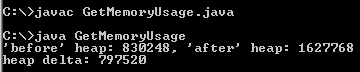# Java Get Memory Usage

If you have prior worked on C or C++ language, you must be aware of that unlike c++, java don't tell that how much memory is occupied by an object defined in the program.### Java Get Memory Usage

If you have prior worked on C or C++ language, you must be aware of that unlike c++, java don't tell that how much memory is occupied by an object defined in the program.# Java Get Memory Usage

If you have prior worked on C or C++ language, you must be aware of that unlike c++,  java don't tell that how much memory is occupied by an object defined in the program.

In this example, we are going to get the used memory from the actual memory. As you know in Java programming memory can be allocated in various forms such as Stack and Heap etc.. you will see that in the given example code output.

Here is the video tutorial of "How to find the Memory Usage in Java?":

Java Code to Get Memory Usage

 ``` public class GetMemoryUsage {   public static void main (String [] args) throws Exception   {   run ();   memoryUsed ();   final int count = 100000;   Object [] object = new Object [count];   long heap1 = 0;   for (int i = -1; i < count; ++ i)   {   Object obj = null;    obj = new Object ();    if (i >= 0)   object [i] = obj;   else   {   obj = null;    run ();   heap1 = memoryUsed ();   }   }   run ();   long heap2 = memoryUsed ();    System.out.println ("'before' heap: " + heap1 +   ", 'after' heap: " + heap2);   System.out.println ("heap delta: " + (heap2 - heap1) );   for (int i = 0; i < count; ++ i) object [i] = null;   object = null;   }  static void run () throws Exception   {   for (int j = 0; j < 4; ++ j) _run ();   }  static void _run () throws Exception   {   long mem1 = memoryUsed (), mem2 = Long.MAX_VALUE;   for (int i = 0; (mem1 < mem2) && (i < 500); ++ i)   {   runtime.runFinalization ();   runtime.gc ();   Thread.currentThread ().yield ();   mem2 = mem1;   mem1 = memoryUsed ();   }   }  static long memoryUsed ()   {   return runtime.totalMemory () - runtime.freeMemory ();   }     static final Runtime runtime = Runtime.getRuntime (); }```

Output will be displayed as: